# Solution assignment 10 Equations with fractions

### Assignment 10

Given the hyperbola: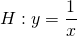and the line: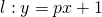For which value(s) of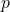is the line tangent to the hyperbola?

### Solution

In order to find the intersection points ofwith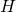, we solve the following equation: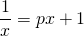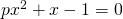Whenis tangent to, this equation has two coinciding points and thus the discriminant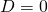: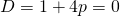Whenhas the value: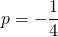the line is tangent to the hyperbola.